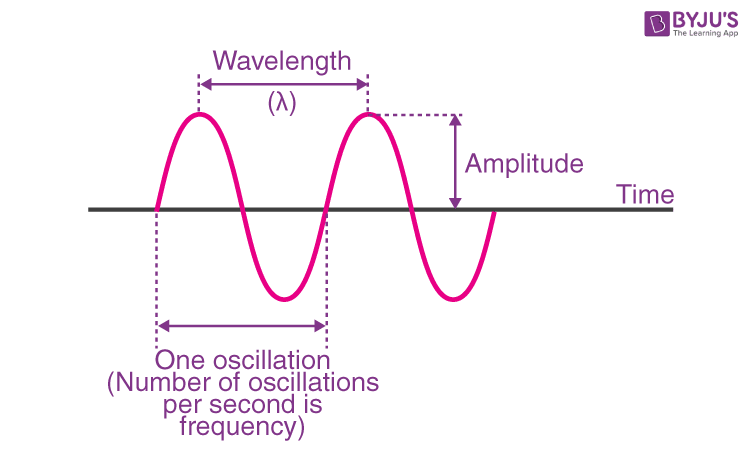# Properties of Waves

Wave is the propagation of disturbance from one place to another in an organised manner. Waves can be mechanical waves or non-mechanical waves. Mechanical waves like sound waves, require a medium to propagate. While electromagnetic waves are non-mechanical waves that do not require a medium and can travel through the vacuum.

If the particles of the medium move in a direction perpendicular to the wave propagation then the waves are called transverse waves. Longitudinal waves are waves in which the particles of the medium vibrate parallel to the direction of wave propagation.## 5 Important Properties of Waves

The main properties of waves are as follows –

Property 1:Amplitude

The maximum displacement of the wave from the mean position is called the amplitude of the wave. It is the maximum height from the centre line to the crest or the trough. The crest is the highest point of the wave and the trough is the lowest point of the wave. Amplitude is measured in metres.

Property 2: Frequency

The number of vibrations passing a fixed point in a given amount of time is called the frequency. The unit of frequency is Hertz.

Property 3: Wavelength

Wavelength is the distance between two identical points (adjacent crests or troughs). It is measured in metres. Frequency and wavelength are inversely proportional to each other.

Property 4: Time Period

The time taken by a complete wave to pass through a particular point is called time period. The time period is measured in seconds. The time period is the reciprocal of the frequency.

Property 5: Speed

For a wave, speed is the distance travelled by a particular point on the wave in the given interval of time. Speed is measured in metres per second.